# PSEB 3rd Class Maths Solutions Chapter 3 Multiplication

Punjab State Board PSEB 3rd Class Maths Book Solutions Chapter 3 Multiplication Textbook Exercise Questions and Answers.

## PSEB Solutions for Class 3 Maths Chapter 3 Multiplication

PAGE 67:

Do you remember?

Fill in the blanks: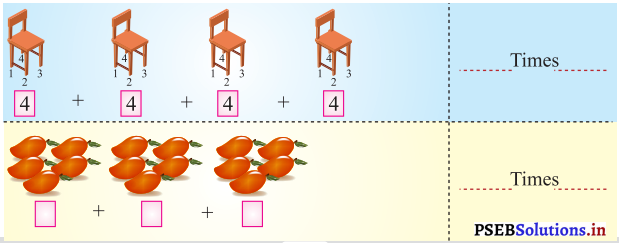Solution.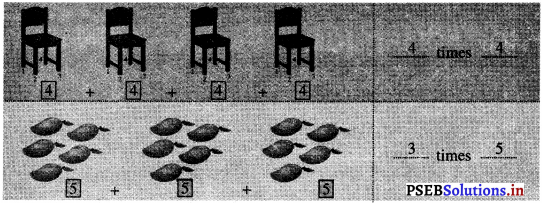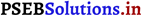PAGE 68:

Question 1.
Avneet Singh has three toy cars. One car has 4 wheels. How many wheels are there in the three cars togcthers?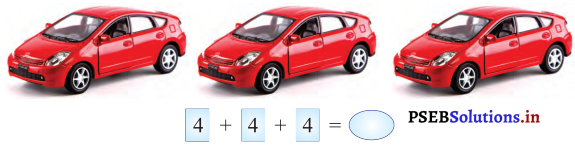Solution.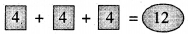Question 2.
If there are 6 children in a class and each child has 5 pencils. How many pencils do all the children have?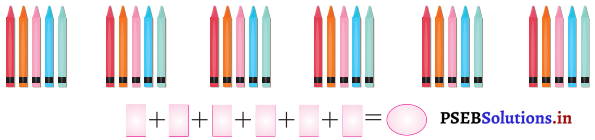Solution.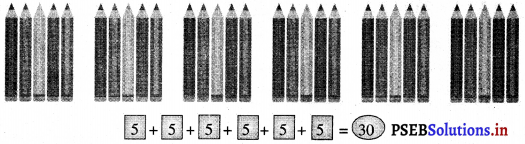Question 3.
Write repeated addition in the form of multiplication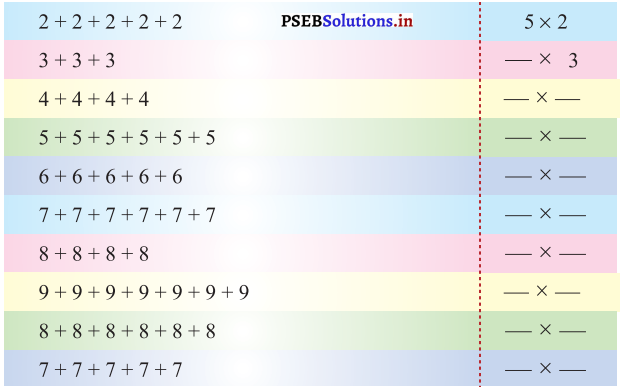Solution.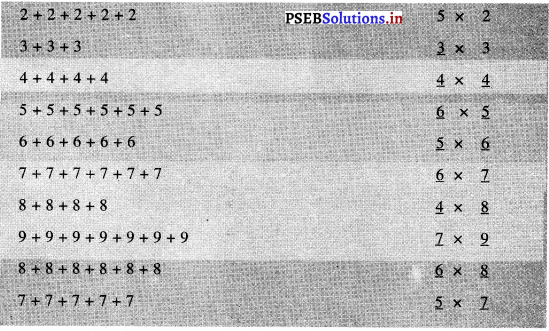PAGE 69: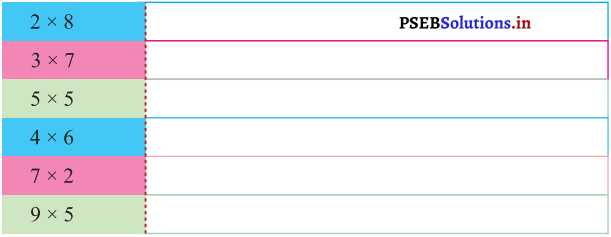Solution.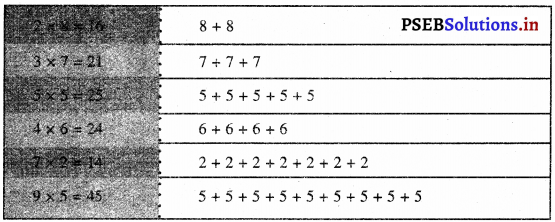PAGE 71:

Let’s do:

Counting in Intervals: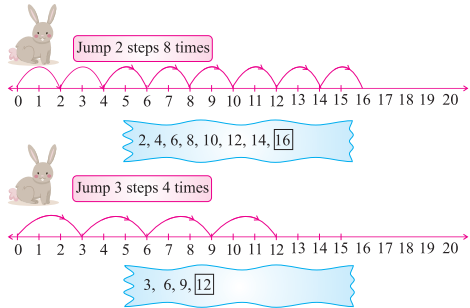Five times jump of four: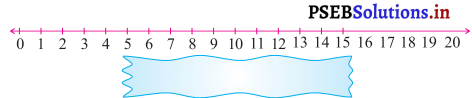Solution.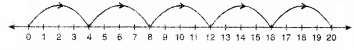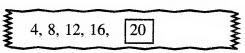Four times jump of five: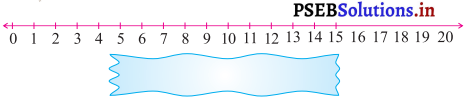Solution.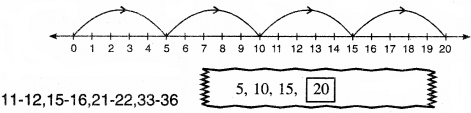PAGE 77:

Let’s do:

Look at the pictures and fill in the blanks.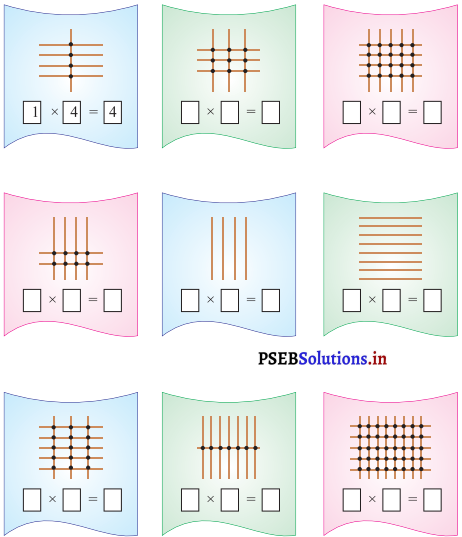Solution.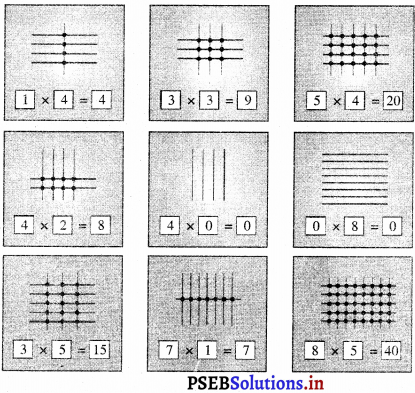PAGE 79:

Let’s do:

write the multiplication table.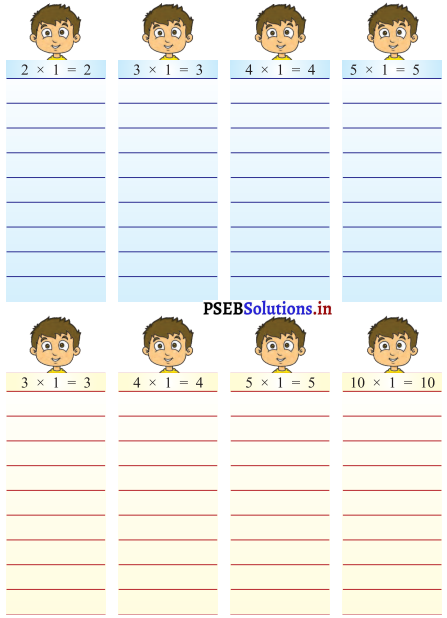Solution.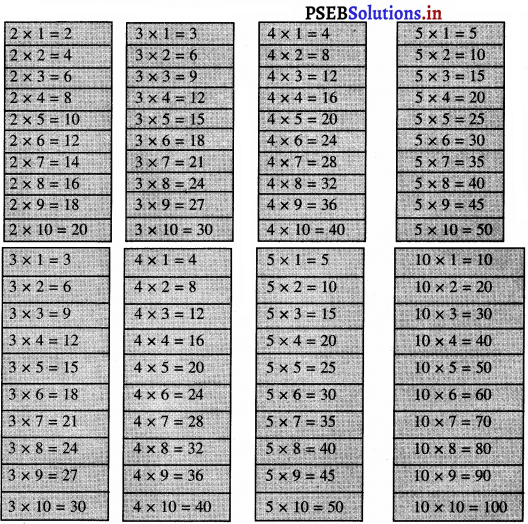PAGE 80:

Word Problems:

In our daily life: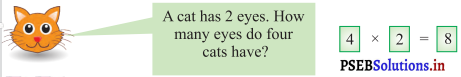a.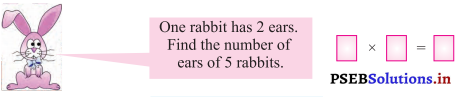Solution.
5 × 2 = 10

b.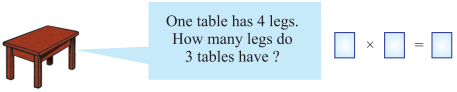Solution.
3 × 4 = 12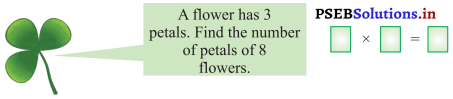Solution.
8 × 3 = 24

d.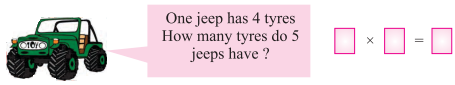Solution.
5 × 4 = 20

e.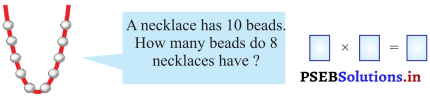Solution.
8 × 10 = 80PAGE 81:

f.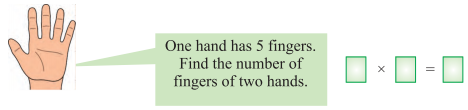Solution.
2 × 5 = 10

g.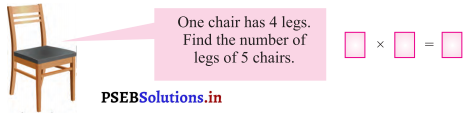Solution.
5 × 4 = 20

h.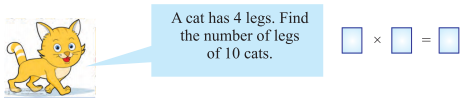Solution.
10 × 4 = 40

i.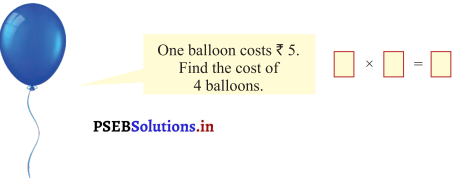Solution.
4 × ₹ 5 = ₹ 20

j.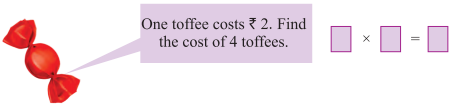Solution.
4 × ₹ 2 = ₹ 8.PAGE 83:
Let’s do:

Question 1.
Fill in the blanks: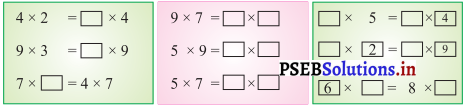Solution.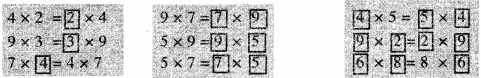Question 2.
Fill in the blanks: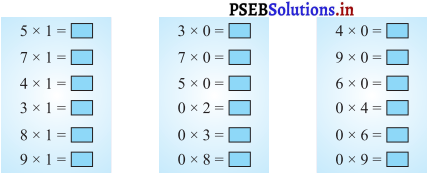Solution.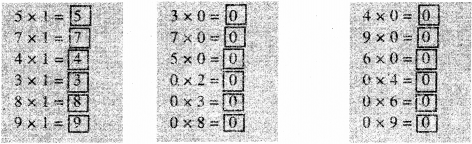Question 3.
Tick (✓) the correct multiples of 2: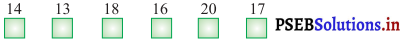Solution.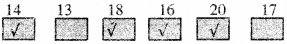Question 4.
Tick (✓) the correct multiples of 5: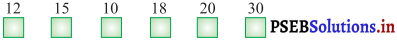Solution.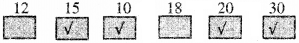PAGE 84:

Fill in the blanks: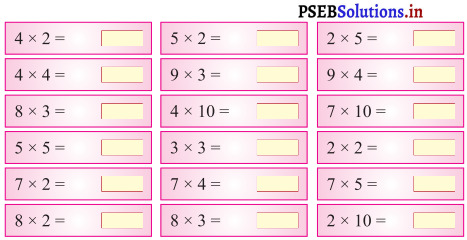Solution.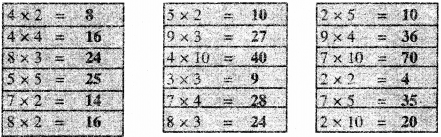Multiply 1-digit with 1-digit number: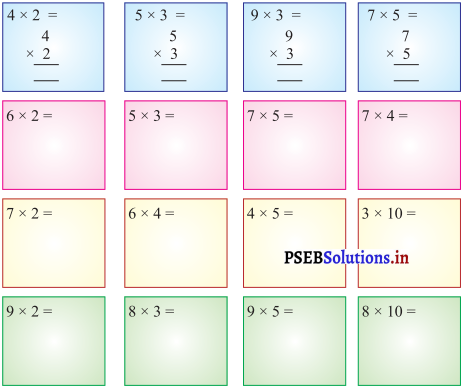Solution.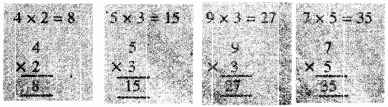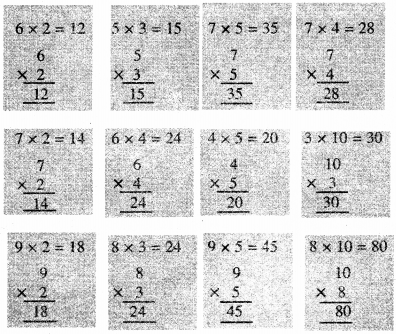PAGE 85:
Let’s do:

Fill in the blanks:

(a) Double of 2 _____
Solution.
4

(b) Double of 3 _____
Solution.
6

(c) Double of 4 _____
Solution.
8

(d) Double of 5 _____
Solution.
10

(e) Double of 6 _____
Solution.
12

(f) Double of 7 _____
Solution.
14

(g) Double of 8 _____
Solution.
16

(h) Double of 9 _____
Solution.
18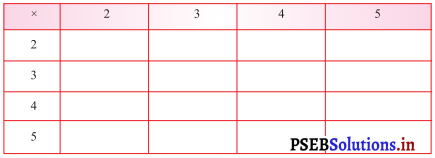Solution.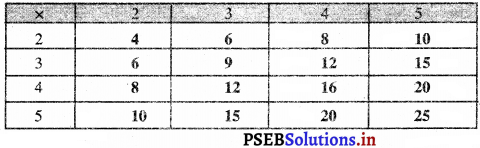PAGE 87:

Let’s count the currency notes of ₹ 10: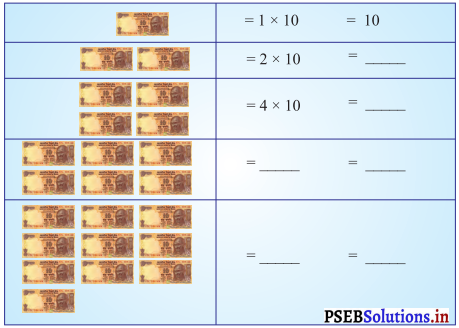Solution.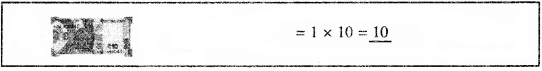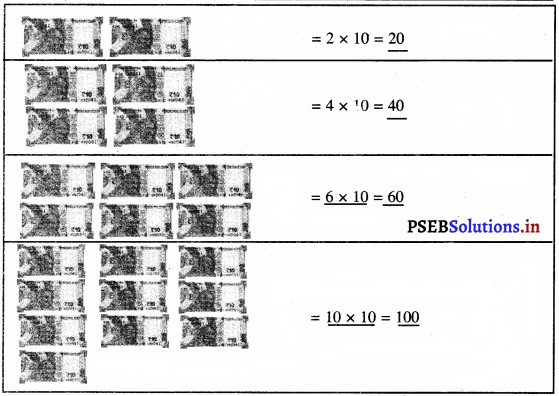Tick (✓) the correct multipies of 10 :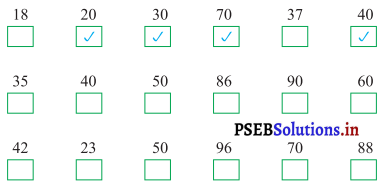Solution.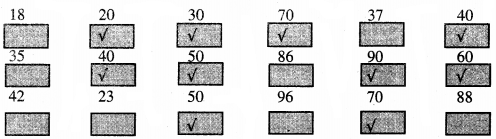Multiples of 10 end with 0.PAGE 88:
Let’s do:

Multiply 2 digit number with 1 digit number.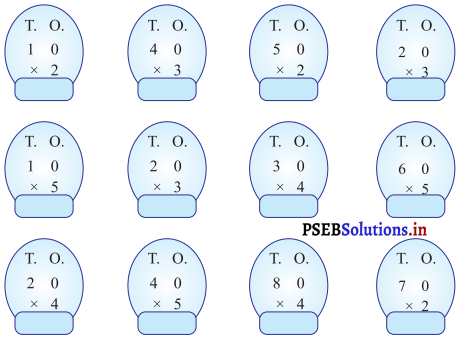Solution.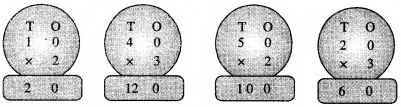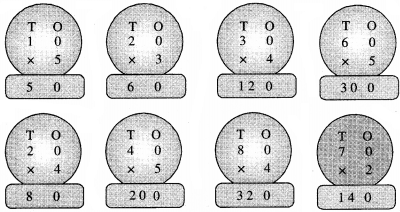PAGE 90:
Let’s do:

Question 1.
One ice cream costs ₹ 55. Find the cost of 5 such ice creams.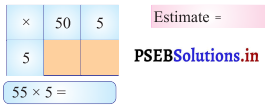Solution.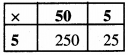Estimate = 275
55 × 5 = 275

Question 2.
A chalk box contains 43 chalks. How many chalks do the 3 boxes have?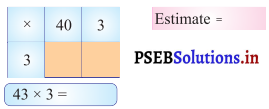Solution.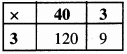Estimate = 129
43 × 3 = 129PAGE 91:
Let’s do:

Multiplication with column method: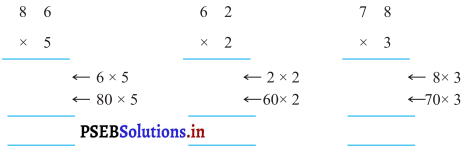Solution.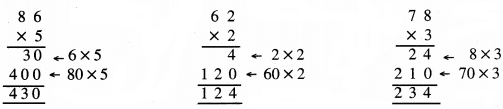PAGE 92:
Let’s do:

Multiplication of 2 digit numbers with 2 digit numbers: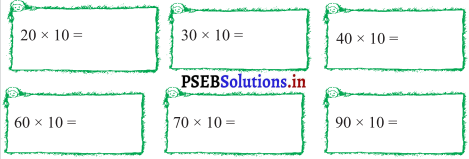Solution.
20 × 10 = 200
30 × 10 = 300
40 × 10 = 400
60 × 10 = 600
70 × 10 = 700
90 × 10 = 900

PAGE 94:
Let’s do: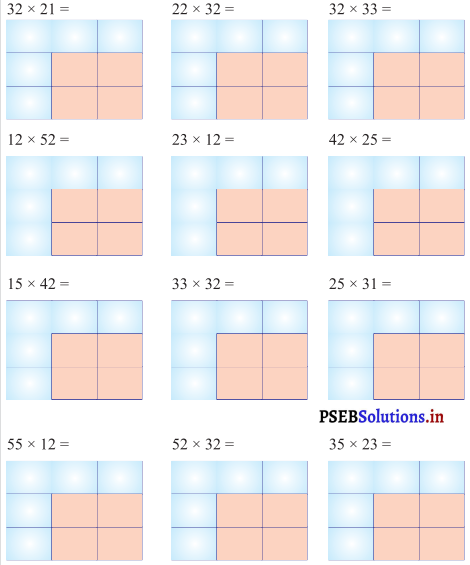Solution.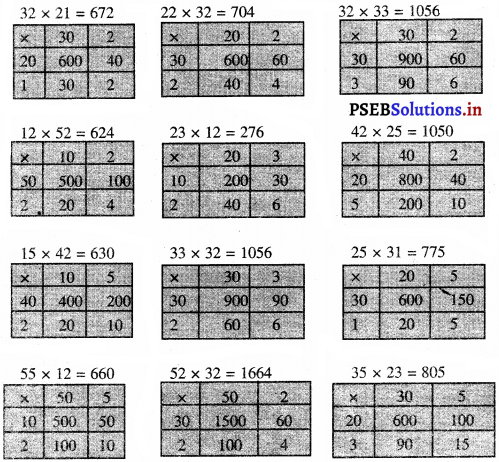PAGE 95:
Let’s do: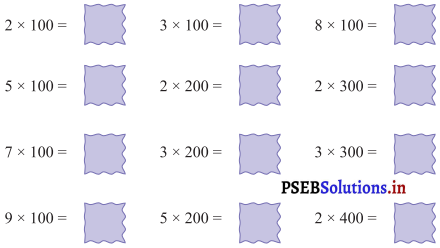Solution.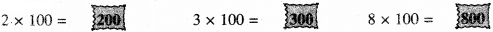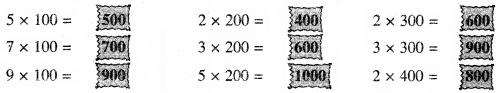Multiplication of 3 digit number with 1 digit number:

PAGE 96:
Let’s do:Solution.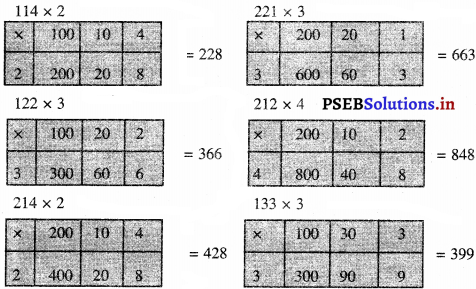PAGE 97:

Lettice Algoritham Method:
Let’s do: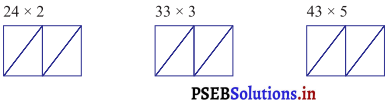Solution.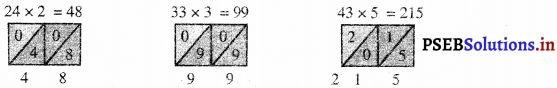PAGE 99:

Worksheet:

Question 1.
Match the columns: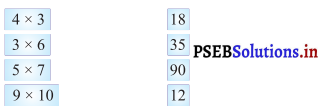Solution.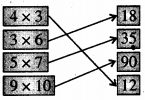Question 2.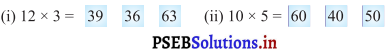Solution.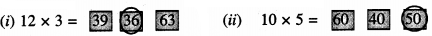Question 3.
Fill in the blanks :
(i) 5 + 5 + 5 + 5 + 5 = ___ × ___
Solution.
5 × 5

(ii) 10 + 10 + 10 + 10 + 10 + 10 + 10 = ___ × ___
Solution.
7 × 10

Question 4.
Fill in the blanks :
(i) 0 × 5 = ___
Solution.
0

(ii) 4 × 1 = ___
Solution.
4

(iii) 3 × 2 = ___ × ___
Solution.
2 × 3Question 5.
Find the product:
(i) 25 × 3 = ___
Solution.
75

(ii) 42 × 14 = ___
Solution.
588

(iii) 70 × 10 = ___
Solution.
700

Question 6.
Fill in the blanks :
(i) 7 × ___ = 21
Solution.
3

(ii) 4 × ___ = 16
Solution.
4

(iii) ___ × 5 = 20
Solution.
4

Question 7.
Write multiplication table of 4 :
4 × 1 = 4
4 × 2 = 8
4 × 3 = 12
4 × 4 = 16
4 × 5 = 20
4 × 6 = 24
4 × 7 = 28
4 × 8 = 32
4 × 9 = 36
4 × 10 = 40Mutilple Choice Questions:

Question 1.
Which number is equal to ten notes of ₹ 10?
(a) 10
(b) 50
(c) 100
(d) 1000
(c) 100.

Question 2.
Which number is equal to five notes of ₹ 10?
(a) 10
(b) 20
(c) 30
(d) 50.